# Mathematics 1010 online

## Linear Equations

An equation is linear if it can be written in the form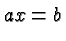where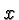is the variable and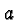and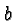are constants. The crucial part of this definition is the phrase can be written, because most linear equations do not occur in as simple a form as above.

### Solutions of a Linear Equation

Let's first ask what kind of solutions are possible for a linear equation. There are three cases:

Clearly there are no other possibilities, and we note the important fact that a linear equation may have none, one, or infinitely many solutions. It is not possible, for example, that a linear equation has two solutions.

The crux of solving a linear equation is to recognize that the equation is linear, and to convert it to the above simple form. In the remainder of this page this process will be illustrated for increasingly complex equations.

• Applying the same operation on both sides of a valid equation gives another valid equation.

• To solve an equation figure out what bothers you and then apply a suitable operation on both sides of the equation to get rid of it.
• Reduce your problem to one you have solved before.

Below you will see several examples of how an equation whose linearity is not obvious can be converted to a clearly linear equation. The examples cover most or all of the techniques required for this class, but of course the list is nowhere near being exhaustive.

• Example 1.We subtract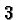andon both sides and obtain the new and equivalent linear equation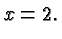• Example 2.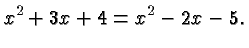We subtract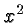on both sides and obtain an equation similar to that in the preceding Example.

• Example 3.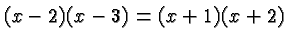We apply the distributive law on both sides and obtain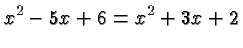which is like the equation in the preceding Example.
• Example 4.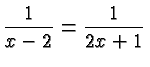The two sides are the same if their reciprocals are the same, and so obtain an equation like that in Example 1: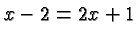• Example 5.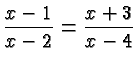Replacing the two sides with their reciprocal does not help in this case. We need to get rid of the denominators. To that end we multiply with both denominators on both sides and obtain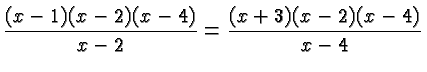Canceling common factors gives an equation like that in Example 3: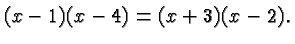Those are the principles, in a nutshell. There are of course a great many more examples and exercises in your textbook.

### Nonlinear Equations

It is important to realize that not all equations are linear. For example, the equation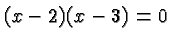has the solutions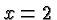and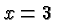, and it is not linear. Since it has two solutions, which is not possible for a linear equation, we can tell without trying that it cannot be converted into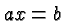.